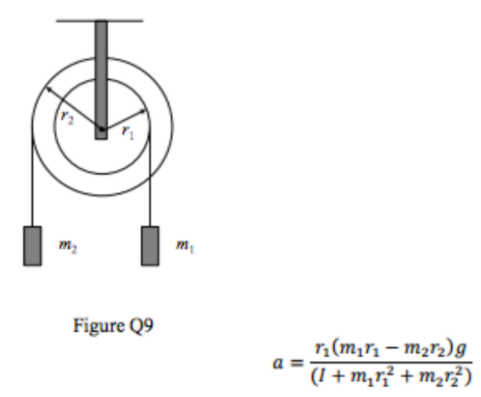# Problem: In the system shown below the double pulley, of moment of inertia I, is fixed to rotate about its centre.a) Draw a free body diagram of the system.b) Write down the equations of motion of the two masses m1 and m2 and the pulley and use these to derive an expression for the initial acceleration of the mass m_1 when the system is released from rest in terms of r1, r2 m1, m2 and I

###### FREE Expert Solution

In this problem, we'll consider the equation of Torque:

$\overline{){\mathbf{\tau }}{\mathbf{=}}{\mathbf{r}}{\mathbf{F}}}$, where F is force and r is the lever arm or the distance where the force is acting.

a)

To draw the free-body diagram for the system, we need to understand the various forces acting on the system.

Let's consider the mass m1 hanging on the rope.

99% (333 ratings)###### Problem Details

In the system shown below the double pulley, of moment of inertia I, is fixed to rotate about its centre.
a) Draw a free body diagram of the system.
b) Write down the equations of motion of the two masses m1 and m2 and the pulley and use these to derive an expression for the initial acceleration of the mass m_1 when the system is released from rest in terms of r1, r2 m1, m2 and I